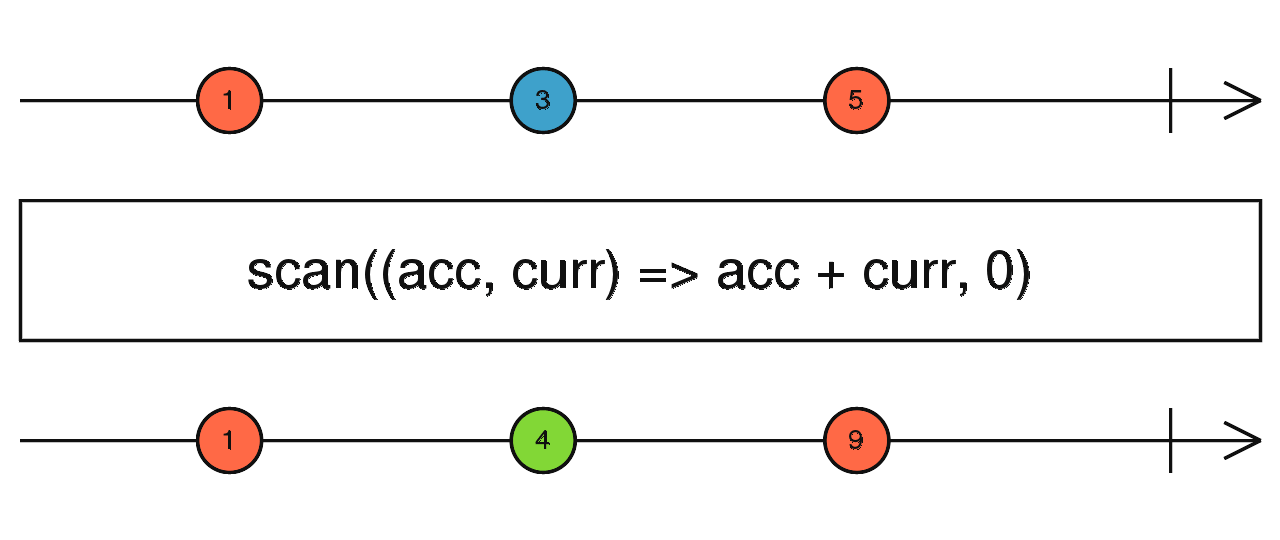/RxJS

# scan

`function` `stable`

Applies an accumulator function over the source Observable, and returns each intermediate result, with an optional seed value.

### `scan<T, R>(accumulator: (acc: R, value: T, index: number) => R, seed?: T | R): OperatorFunction<T, R>`

#### Parameters

 accumulator The accumulator function called on each source value. seed Optional. Default is `undefined`. The initial accumulation value.

#### Returns

`OperatorFunction<T, R>`: An observable of the accumulated values.

## Description

It's like `reduce`, but emits the current accumulation whenever the source emits a value.Combines together all values emitted on the source, using an accumulator function that knows how to join a new source value into the accumulation from the past. Is similar to `reduce`, but emits the intermediate accumulations.

Returns an Observable that applies a specified `accumulator` function to each item emitted by the source Observable. If a `seed` value is specified, then that value will be used as the initial value for the accumulator. If no seed value is specified, the first item of the source is used as the seed.

## Example

Count the number of click events

```import { fromEvent } from 'rxjs';
import { scan, mapTo } from 'rxjs/operators';

const clicks = fromEvent(document, 'click');
const ones = clicks.pipe(mapTo(1));
const seed = 0;
const count = ones.pipe(scan((acc, one) => acc + one, seed));
count.subscribe(x => console.log(x));```

### `scan(accumulator: (acc: R, value: T, index: number) => R, seed: R): OperatorFunction<T, R>`

#### Parameters

 accumulator Type: `(acc: R, value: T, index: number) => R`. seed Type: `R`.

#### Returns

`OperatorFunction<T, R>`

### `scan(accumulator: (acc: T, value: T, index: number) => T, seed?: T): MonoTypeOperatorFunction<T>`

#### Parameters

 accumulator Type: `(acc: T, value: T, index: number) => T`. seed Optional. Default is `undefined`. Type: `T`.

#### Returns

`MonoTypeOperatorFunction<T>`

### `scan(accumulator: (acc: R, value: T, index: number) => R): OperatorFunction<T, R>`

#### Parameters

 accumulator Type: `(acc: R, value: T, index: number) => R`.

#### Returns

`OperatorFunction<T, R>`